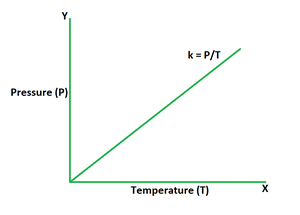Open in App
Not now

# Gay Lussac’s Law Formula

• Last Updated : 01 May, 2022

Gay Lussac law relates the values of pressure and temperature of a gas. It states that for a gas with a fixed mass held at a constant volume, the ratio of initial pressure and temperature is equal to the ratio of final pressure and temperature. From the given law statement, it can be interpreted that the pressure produced by a gas is directly proportional to its temperature if mass and volume are kept fixed. To put it another way, the pressure exerted by a gas (with a given mass and constant volume) changes directly with its absolute temperature.Formula

P1/T1 = P2/T2

where,

P1 is the initial pressure,

T1 is the initial temperature,

P2 is the final pressure,

T2 is the final temperature.

Derivation

It is known that the pressure of a gas is proportional to its temperature. This relation can be written as,

P ∝ T

Replacing the proportionality sign with constant k we get,

P = kt

The above equation is valid for all values of pressure and temperature.

Suppose a gas is exerting pressure P1 at a temperature T1. The equation that defines this situation is,

P1 = kT1

k = P1/T1     ……… (1)

The temperature of the gas changes to T2, which changes the pressure to P2. This can be expressed as,

P2 = kT2

k = P2/T2     ……… (2)

From (1) and (2) we get,

P1/T1 = P2/T2

This derives the Gay Lussac law for gases.

Examples of Gay Lussac’s Law

1. Increasing the heat to a pressure cooker increases the pressure inside it. This results in less cooking time as the steam does not lose taste of food to the air due to the sealed container.

2. If too much air is put in vehicle tyres, they may over-pressurize due to high temperature. But on a cold day, their pressure reduces which results in underinflation.

3. Aerosol cans shouldn’t be kept in high temperatures because heat raises the pressure inside them which may lead to explosion.

### Sample Problems

Problem 1. The initial pressure of a gas inside a tank is 2 Pa at 100 K. Find the final pressure if the gas is heated to a temperature of 200K.

Solution:

We have,

P1 = 2

T1 = 100

T2 = 200

Using the formula we get,

P1/T1 = P2/T2

=> 2/100 = P2/200

=> P2/200 = 1/50

=> P2 = 200/50

=> P2 = 4 Pa

Problem 2. The initial pressure of a gas inside a tank is 3 Pa at 120 K. Find the final pressure if the gas is cooled to a temperature of 60K.

Solution:

We have,

P1 = 3

T1 = 120

T2 = 60

Using the formula we get,

P1/T1 = P2/T2

=> 3/120 = P2/60

=> P2/60 = 1/40

=> P2 = 60/40

=> P2 = 1.5 Pa

Problem 3. The final pressure of a gas inside a tank is 12 Pa at 200 K. Find the initial pressure if the initial temperature of gas was 50 K.

Solution:

We have,

P2 = 12

T1 = 50

T2 = 200

Using the formula we get,

P1/T1 = P2/T2

=> P1/50 = 12/200

=> P1/50 = 3/50

=> P1 = 3 Pa

Problem 4. The final pressure of a gas inside a tank is 4 Pa at 300 K. Find the initial pressure if the initial temperature of gas was 600 K.

Solution:

We have,

P2 = 4

T1 = 600

T2 = 300

Using the formula we get,

P1/T1 = P2/T2

=> P1/600 = 4/300

=> P1/600 = 1/75

=> P1 = 600/75

=> P1 = 8 Pa

Problem 5. The initial pressure of a gas inside a tank is 2 Pa at 300 K. Find the final temperature of gas which increased its pressure value to 8 Pa.

Solution:

We have,

P1 = 2

P2 = 8

T1 = 300

Using the formula we get,

P1/T1 = P2/T2

=> 2/300 = 8/T2

=> T2/8 = 300/2

=> T2/8 = 150

=> T2 = 1200 K

Problem 6. The initial pressure of a gas inside a tank is 10 Pa at 150 K. Find the final temperature of gas which decreased its pressure value to 5 Pa.

Solution:

We have,

P1 = 10

P2 = 5

T1 = 150

Using the formula we get,

P1/T1 = P2/T2

=> 10/150 = 5/T2

=> T2/5 = 150/10

=> T2/5 = 15

=> T2 = 75 K

Problem 7. The final pressure of a gas inside a tank is 15 Pa at 900 K. Find the initial temperature if the initial pressure of gas was 3 Pa.

Solution:

We have,

P1 = 3

P2 = 15

T2 = 900

Using the formula we get,

P1/T1 = P2/T2

=> 3/T1 = 15/900

=> T1/3 = 900/15

=> T1/3 = 60

=> T1 = 180 K

My Personal Notes arrow_drop_up